Next: The secant equation Up: Annexes Previous: Gram-Schmidt orthogonalization procedure.   Contents

# Notions of constrained optimization

Let us define the problem:
Find the minimum of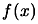subject to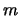constraints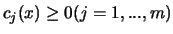.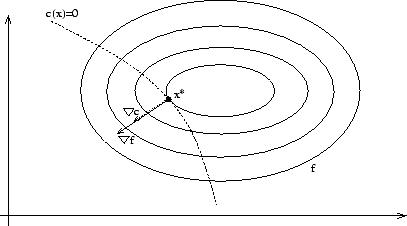To be at an optimum point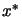we must have the equi-value line (the contour) oftangent to the constraint border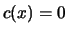. In other words, when we have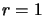constraints, we must have (see illustration in Figure 13.2) (the gradient of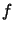and the gradient ofmust aligned):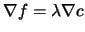In the more general case when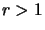, we have: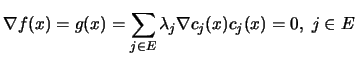(13.19)

Where E is the set of active constraints, that is, the constraints which have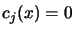We define Lagrangian function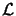as: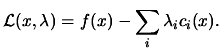(13.20)

The Equation 13.19 is then equivalent to: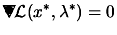where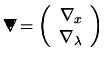(13.21)

In unconstrained optimization, we found an optimumwhen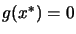. In constrained optimization, we find an optimum point (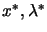), called a KKT point (Karush-Kuhn-Tucker point) when: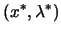is a KKT point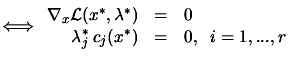(13.22)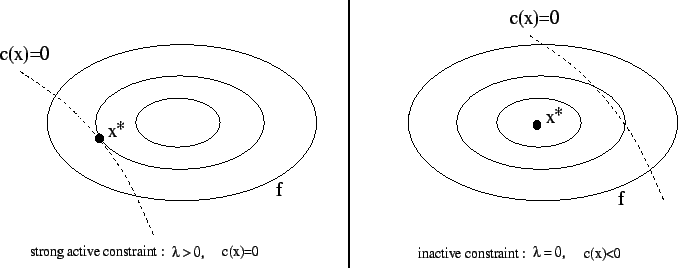The second equation of 13.22 is called the complementarity condition. It states that both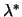and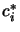cannot be non-zero, or equivalently that inactive constraints have a zero multiplier. An illustration is given on figure 13.3.
To get an other insight into the meaning of Lagrange Multipliers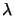, consider what happens if the right-hand sides of the constraints are perturbated, so that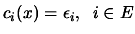(13.23)

Let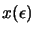,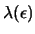denote how the solution and multipliers change as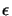changes. The Lagrangian for this problem is: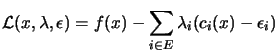(13.24)

From 13.23,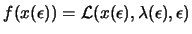, so using the chain rule, we have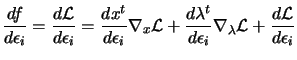(13.25)

Using Equation 13.21, we see that the terms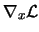and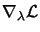are null in the previous equation. It follows that: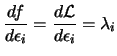(13.26)

Thus the Lagrange multiplier of any constraint measure the rate of change in the objective function, consequent upon changes in that constraint function. This information can be valuable in that it indicates how sensitive the objective function is to changes in the different constraints.Next: The secant equation Up: Annexes Previous: Gram-Schmidt orthogonalization procedure.   Contents
Frank Vanden Berghen 2004-04-19